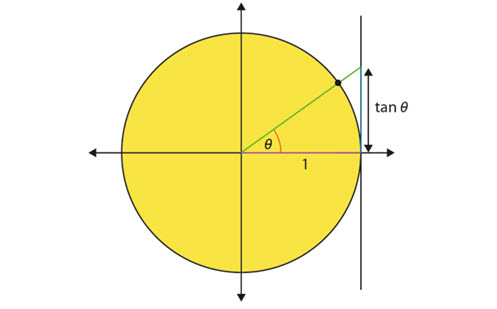# Trigonometry (Years 9 and 10)

This paper introduces trigonometry by breaking it into two sections:

• Understanding trigonometric functions
• Using trigonometry to solve problems in context.

It provides details of the knowledge students should have and some of the misconceptions they may hold. Examples are given to illustrate what these misconceptions may look like, how to address them, and support teachers in developing pedagogical and content knowledge.

Year level(s) Year 9, Year 10
Purpose Content knowledge
Keywords Trigonometry, ratio, sine, cosine, tangent

## Curriculum alignment

Strand and focus Geometry
Topics Pythagoras and trigonometry
AC: Mathematics content descriptions
ACMMG223 Use similarity to investigate the constancy of the sine, cosine and tangent ratios for a given angle in right-angled triangles
ACMMG224 Apply trigonometry to solve right-angled triangle problems
ACMMG245 Solve right-angled triangle problems including those involving direction and angles of elevation and depression